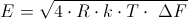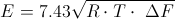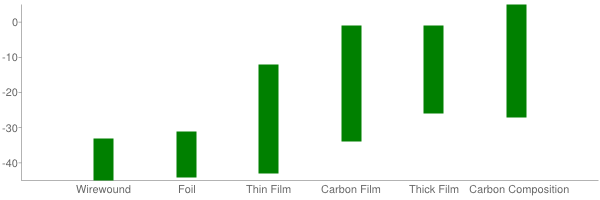# Resistor Noise

## What is resistor noise?

Noise is an unwanted phenomenon for resistors. For some applications the noise properties are important. Examples are high gain amplifiers, charge amplifiers and low-level signals. Resistor noise is often specified as microvolts noise per volt of applied voltage, for a 1 MHz bandwidth. Thermal noise is the predominant source of noise for resistors.  It is dependent on three variables: resistance, temperature and bandwidth. The relation between these three parameters is describes by the formula:Where E is the RMS noise signal in volts, R is the resistance in ohms, k is Boltzmann’s constant, T is the temperature in Kelvin and dF is the bandwidth in Hz. The equation shows that the noise level can be decreased by reducing the resistance, the temperature or the bandwidth. Knowing Boltzmann’s constant, the formula is simplified to:Where E is now the noise voltage in nanovolts, R in kΩ, and dF in kHz.

## Thermal and current noise

There are two types of noise: the thermal noise and the current noise. To understand their principle, they will be discussed in more detail. In all materials, the electrons permanently move. As temperature increases, the movements increase. The vibrations of the electrons cause an electric signal (AC) across the terminals of the component. Because the vibrations are completely random, the electrical signal is noise. This is called thermal noise or Johnson noise. It is the main contributor to noise for resistors. Thermal noise is constant over a wide frequency range. Current noise however, declines when frequency is increased. The thermal noise increases with a larger resistance value, while the current noise decreases.

## Noise standards

The way to measure resistor current noise is defined in norm IEC 60195. This makes the comparison of different manufacturers possible. The current noise of a resistor is described by the current noise index with a code number.

## Low Noise Resistor

Thin film, metal foil and wire wound resistors have better noise characteristics than other types. Therefore they are often specified in low-noise amplifying applications. The carbon composition resistor and thick film are of the worst types. They cope with high noise due to the construction and material.Noise index (dB) for the main resistor types

## Resistor applications

In every amplifier circuit, the input resistor is critical. Any noise at the input signal will be amplified to the full gain. It is therefore of high importance to choose a low-noise resistor at the first stage, as well as a low resistance value. This is however not valid for a load resistor, since the gain that is obtained from a high resistance value outweighs the higher noise level. Because thermal noise is temperature dependent, it is very effective to cool the input stages to reach a low-noise performance.